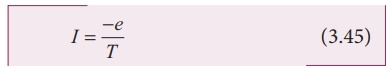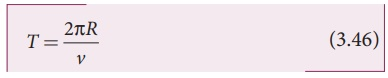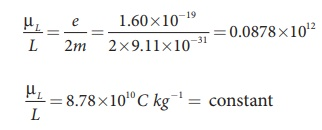Home | | Physics 12th Std | Magnetic dipole moment of revolving electron

# Magnetic dipole moment of revolving electron

The circulating electron in a loop is like current in a circular loop (since flow of charge is current).

Magnetic dipole moment of revolving electron

Suppose an electron undergoes circular motion around the nucleus as shown in Figure 3.41. The circulating electron in a loop is like current in a circular loop (since flow of charge is current). The magnetic dipole moment due to current carrying circular loop isIn magnitude,

µL = I AIf T is the time period of an electron, the current due to circular motion of the electron iswhere −e is the charge of an electron. If R is the radius of the circular orbit and v is the velocity of the the velocity of the electron in the circular orbit, thenUsing equation (3.45) and equation (3.46) in equation (3.44), we getwhere A = πR2 is the area of the circular loop. By definition, angular momentum of the electron about O isUsing equation (3.47)  and  equation (3.48), we getThe negative sign indicates that the magnetic moment and angular momentum are in opposite direction.

In magnitude,The ratio µL/L is a constant and also known as gyro-magnetic ratio (e/2m). It must be noted that the gyro-magnetic ratio is a constant of proportionality which connects angular momentum of the electron and the magnetic moment of the electron.

According to Neil’s Bohr quantization rule, the angular momentum of an electron moving in a stationary orbit is quantized, which means,where, h is the Planck’s constant (h = 6.63 x 10-34 J s ) and number n takes natural numbers

(i.e., n = 1,2,3,....). Hence,The minimum magnetic moment can be obtained by substituting n = 1,where, µB =eh/4πm= 9.27 ×10−24 Am2  is called Bohr magneton. This is a convenient unit with which one can measure atomic magnetic moments.

Note: Bohr quantization rule will be discussed in unit 8 of second volume

Tags : Biot - Savart Law | Physics , 12th Physics : Magnetism and Magnetic Effects of Electric Current
Study Material, Lecturing Notes, Assignment, Reference, Wiki description explanation, brief detail
12th Physics : Magnetism and Magnetic Effects of Electric Current : Magnetic dipole moment of revolving electron | Biot - Savart Law | Physics